# Discrete Data

Discrete Data

In statistics, quantitative data is categorised into two types- discrete data and continuous data.

Carefully observe the image given below and try to interpret the meaning of discrete and continuous data.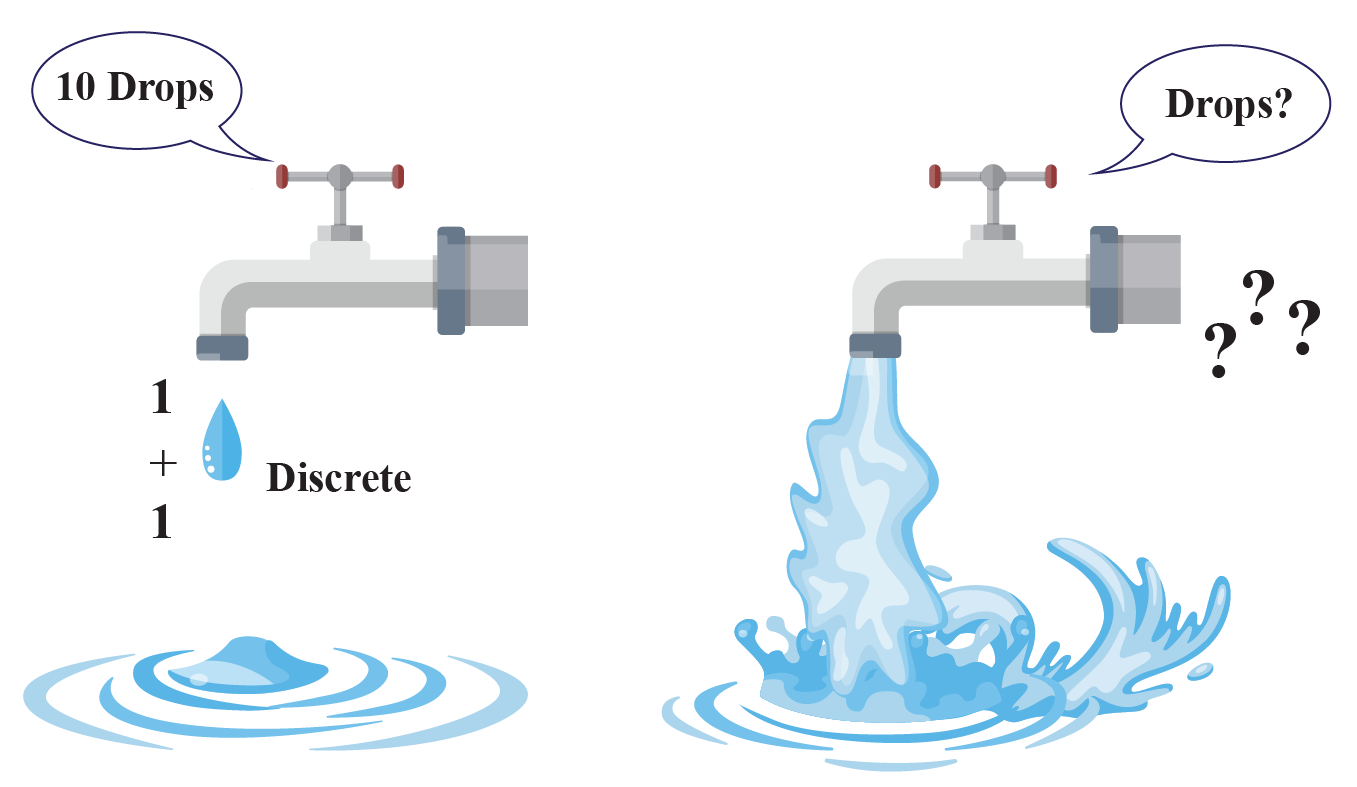Let's explore further.

In this mini-lesson, we will learn about discrete data definition, difference between discrete and continuous data and graphical representation of discrete data through visualization, some solved examples and a few interactive questions for you to test your understanding.

## Lesson Plan

 1 What Is Discrete Data? 2 Tips and Tricks 3 Important Notes on Discrete Data 4 Solved Examples on Discrete Data 5 Interactive Questions on Discrete Data

## What Is Discrete Data?

The word data came from the Latin word 'Datum' which means “something given”.

After a research question is developed, data is being collected continuously through observation. Then it is organized, summarized, classified, and then represented graphically.

Data can be divided into two categories- Qualitative data and Quantitative data.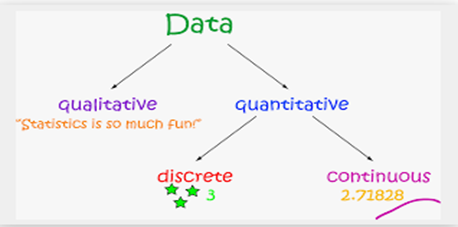Among these two categories, quantitative data can be further classified into two types, discrete and continuous data. Now, let us look at discrete data definition.

When values in a data set are countable and can only take certain values, it is called discrete data. For example, number of students in a class, number of players required in a team, etc.

We can easily count the variables in a discrete data.

Now, let us learn about the difference between discrete and continuous data in the next section.

## What Is the Difference Between Discrete and Continuous Data?

Let us understand the difference between discrete data v/s. continuous data through the following table:

 Discrete Data Continuous Data Variables can take only specific values. Variables can take any value within a range. We can count the values in a discrete data series. We cannot count but measure the values in a continuous data series. Whole numbers are used to write discrete data values. Real numbers are used to used to write continuous data values.

Examples

Some of the examples of discrete and continuous data are given in the table below:

 Discrete data Continuous data Number of books in a bookshelfLength of pages of books present in a bookshelfNumber of players participated in a raceTime taken by each player to finish the raceNumber of students present in a classWeight of each student in a class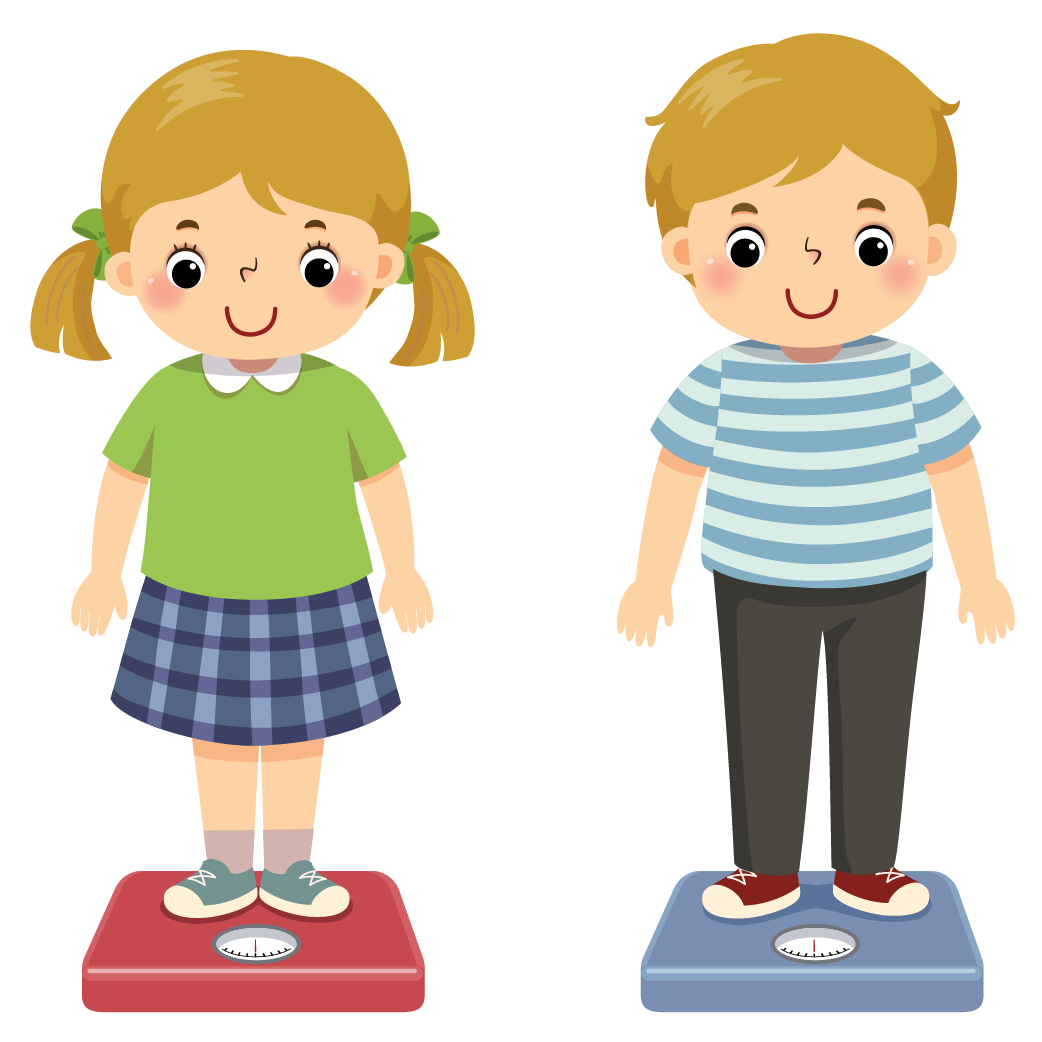Tips and Tricks
1. Whenever you have to identify, whether a given data is discrete or not, just ask yourself a question:

"Can I count it?"

## Graphical Representation of Discrete Data

Following types of graph can be used for graphical representation of discrete data:

• Bar Graph
• Frequency table
• Line plot(number line)

Bar graph is the most suitable way to represent discrete data, as finite values can be presented clearly through vertical or horizontal bars.

In frequency table, values are represented through tally marks and frequency of each variable.

On number line, we marked the value of each variable on the number line.

Let us take an example to understand it better.

Examples

In a survey with 14 children on their favourite ice-cream flavour, it was found that 4 children like butterscotch flavour, 5 children like chocolate flavour, 3 children like vanilla flavour and 2 children like strawberry flavour of ice-cream.

It is an example of discrete data as we can count the number of children who like a particular flavour of ice-cream.

Carefully observe the graphical representation of this data through all three modes of representation.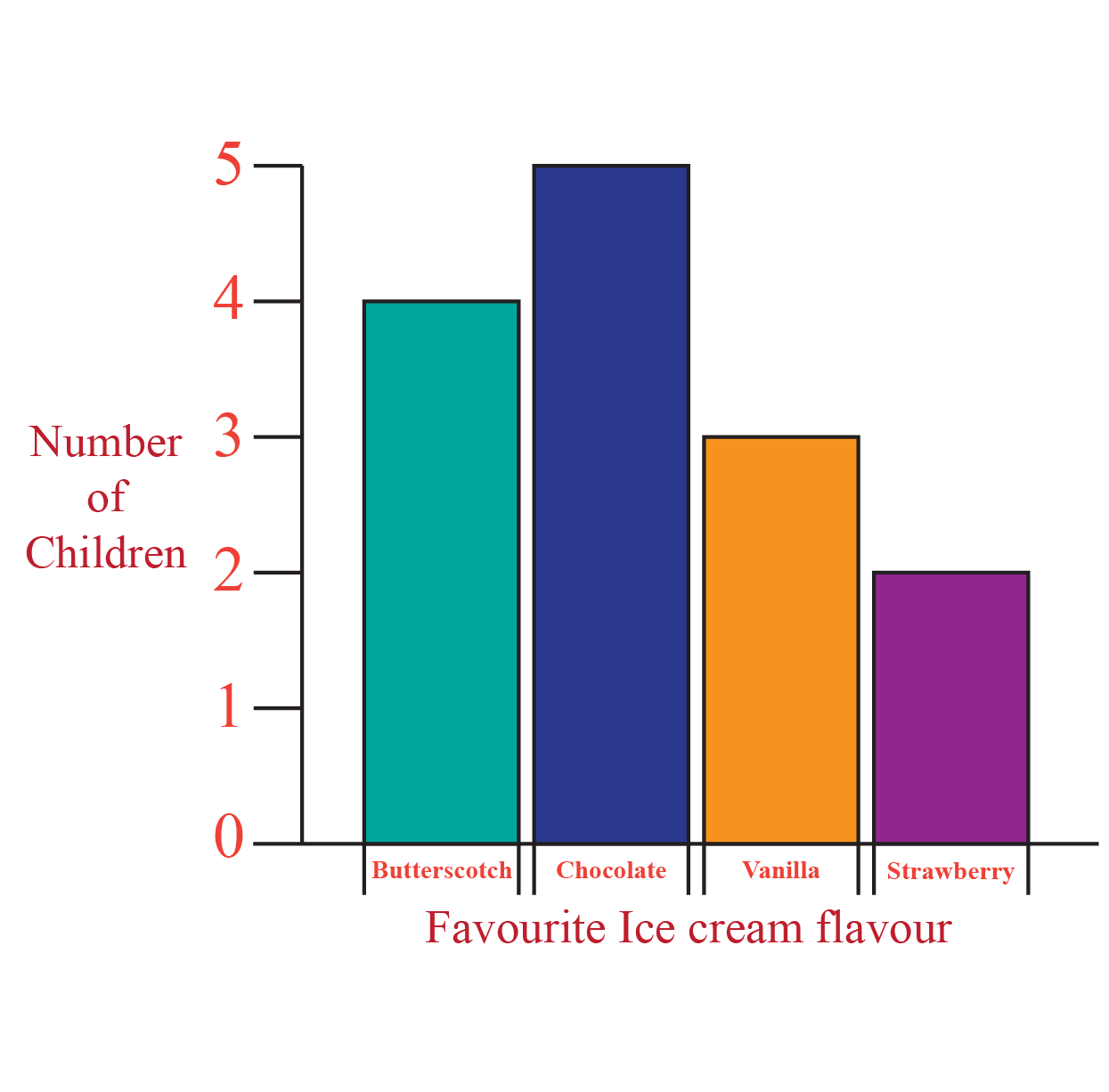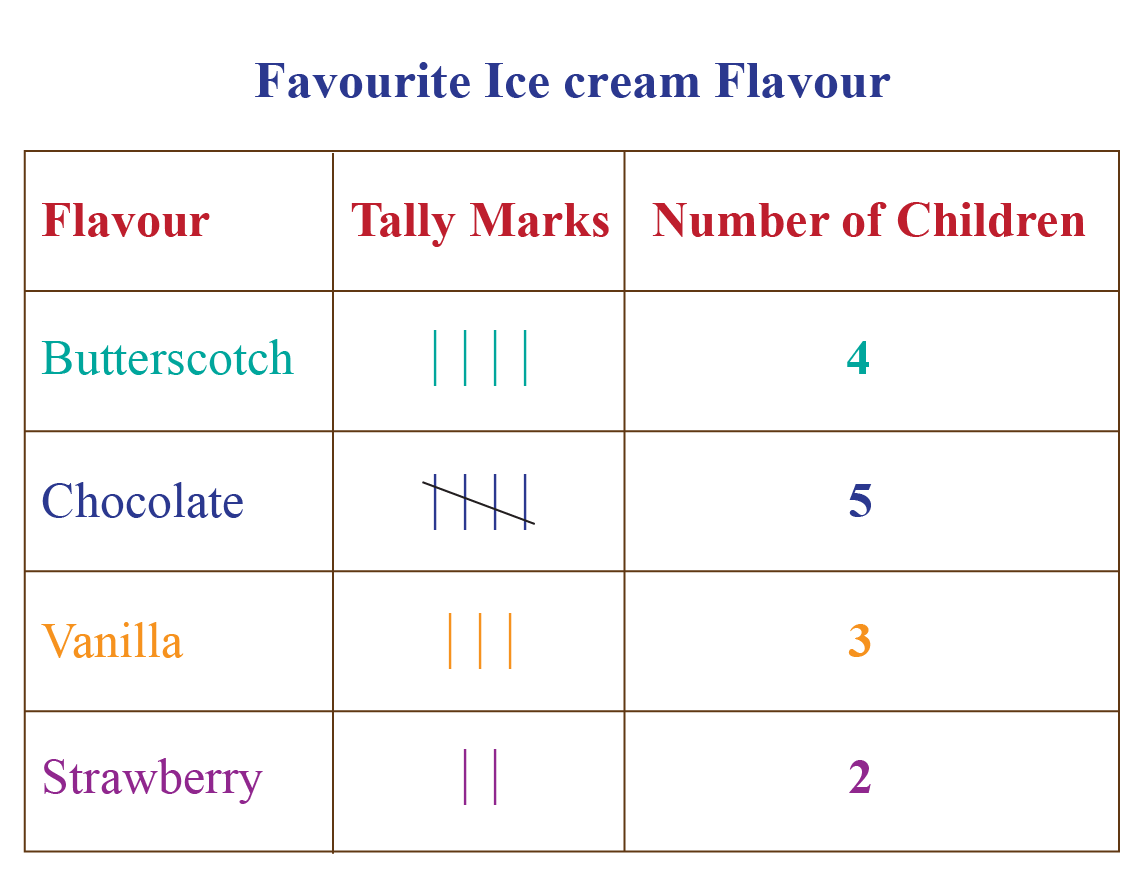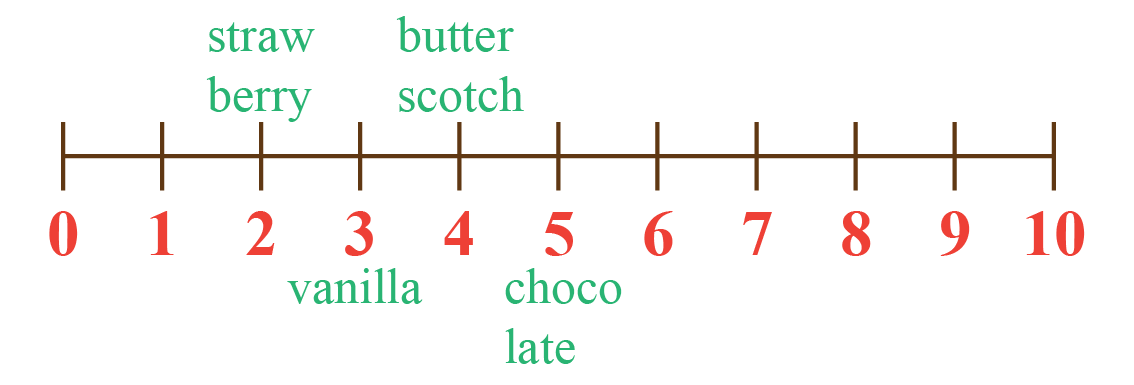More Important Topics
More Important Topics
Learn from the best math teachers and top your exams

• Live one on one classroom and doubt clearing
• Practice worksheets in and after class for conceptual clarity
• Personalized curriculum to keep up with school